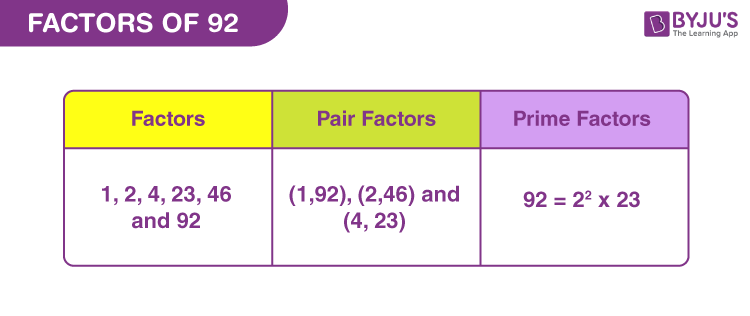# Factors of 92

Factors of 92 are the real numbers that can divide the original number, completely. If ‘n’ is the factor, then ‘n’ divides 92 into equal parts. For example, if 7 is the factor of 21, then 21 divided by 7 is equal to 3. Thus, 7 divides 21 into three equal parts. Also, we need to observe, there is no remainder left after division and the quotient, produced, is a whole number.

21 ÷ 7 = 3 [Remainder = 0 and quotient = 3]

Similarly, the factors of 92, divide it into equal parts. Here, we will calculate the factors along with pair factors and prime factors.## How to Find Factors of 92?

Factors of 92 are the integers that divide the original number, evenly. 92 is a composite number, therefore, it has more than two factors. Thus, all the factors will divide 92 into equal parts.

To find the factors, we need to start dividing 92 by the smallest natural number, i.e., 1.

92 ÷ 1 = 92

92 ÷ 2 = 46

92 ÷ 4 = 23

92 ÷ 23 = 4

92 ÷ 46 = 2

92 ÷ 92 = 1

Thus, the factors of 92 are 1, 2, 4, 23, 46 and 92.

## Pair Factors of 92

The product of pair factors will result in the original number. Thus, to find the pair factors of 92, we need to multiply two such factors, the product of which results in 92.

1 × 92 = 92

2 × 46 = 92

4 × 23 = 92

Therefore, the pair factors are (1, 92), (2, 46) and (4, 23).

As we can see, these were the positive pair factors. Similarly, we can also consider negative pair factors that will result in the original number.

-1 × -92 = 92

-2 × -46 = 92

-4 × -23 = 92

Therefore, the negative pair factors are (-1, -92), (-2, -46) and (-4, -23).

## Prime Factorisation of 92

Prime factors of 92 divide the original number into equal parts. These are prime numbers that can be determined by the prime factorization method.

According to prime factorisation, we have to divide 92, by the prime numbers, until we get 1 as the dividend.

Step 1: 92 is an even number, so it can be divided by the smallest prime number, i.e.,2

92/2 = 46

Step 2: Again 46 is an even number and divisible by 2

46/2 = 23

Step 3: 23 is itself a prime number, therefore, it is divisible by 23 only.

23/23 = 1

Therefore,

 Prime factorisation of 92 = 2 x 2 x 23 = 22 x 23

## Solved Examples

Q.1: There are 92 coins in a piggy bank. Nisha wants to divide these coins and put into 4 separate piggy bank. How many coins does each piggy bank hold at the end?

Solution: Given,

Number of coins = 92

Number of piggy bank = 4

Number of coins in each piggy bank = 92/4 = 23

Q.2: Find the sum of all the factors of ninety-two.

Solution: The factors of 92 are 1, 2, 4, 23, 46 and 92.

Sum = 1 + 2 + 4 + 23 + 46 + 92 = 168

Therefore, 168 is the required sum.

Q.3: What is the greatest common factor of 42 and 92?

Answer: Let us write the factors of both the numbers.

42 → 1, 2, 3, 6, 7, 14, 21, 42

92 → 1, 2, 4, 23, 46 and 92

Hence, the greatest common factor is 2.

## Frequently Asked Questions on Factors of 92

### 1. What are the factors of 92?

Answer: There are a total of six factors of 92, they are 1, 2, 4, 23, 46 and 92.

### 2. What are the multiples of 92?

Answer: The first 10 multiples of 92 are 92, 184, 276, 368, 460, 552, 644, 736, 828 and 920.

### 3. Is 92 a prime or composite?

Answer: 92 is a composite number because it has more than two factors.

### 4. What is 92 as a product of primes?

Answer: The prime factorization of 92 is given by:

92 = 2 x 2 x 23 or 22 x 23

### 5. The product of which two factors produces 92?

Answer: The pair factors, whose products produce 92 are (1, 92), (2, 46) and (4, 23)

### 6. What is the GCF of 92 and 23?

Answer: GCF of 92 and 23 is 23.

23 → 1, 23

92 → 1, 2, 4, 23, 46, 92

Hence, 23 is the greatest common factor of 23 and 92.